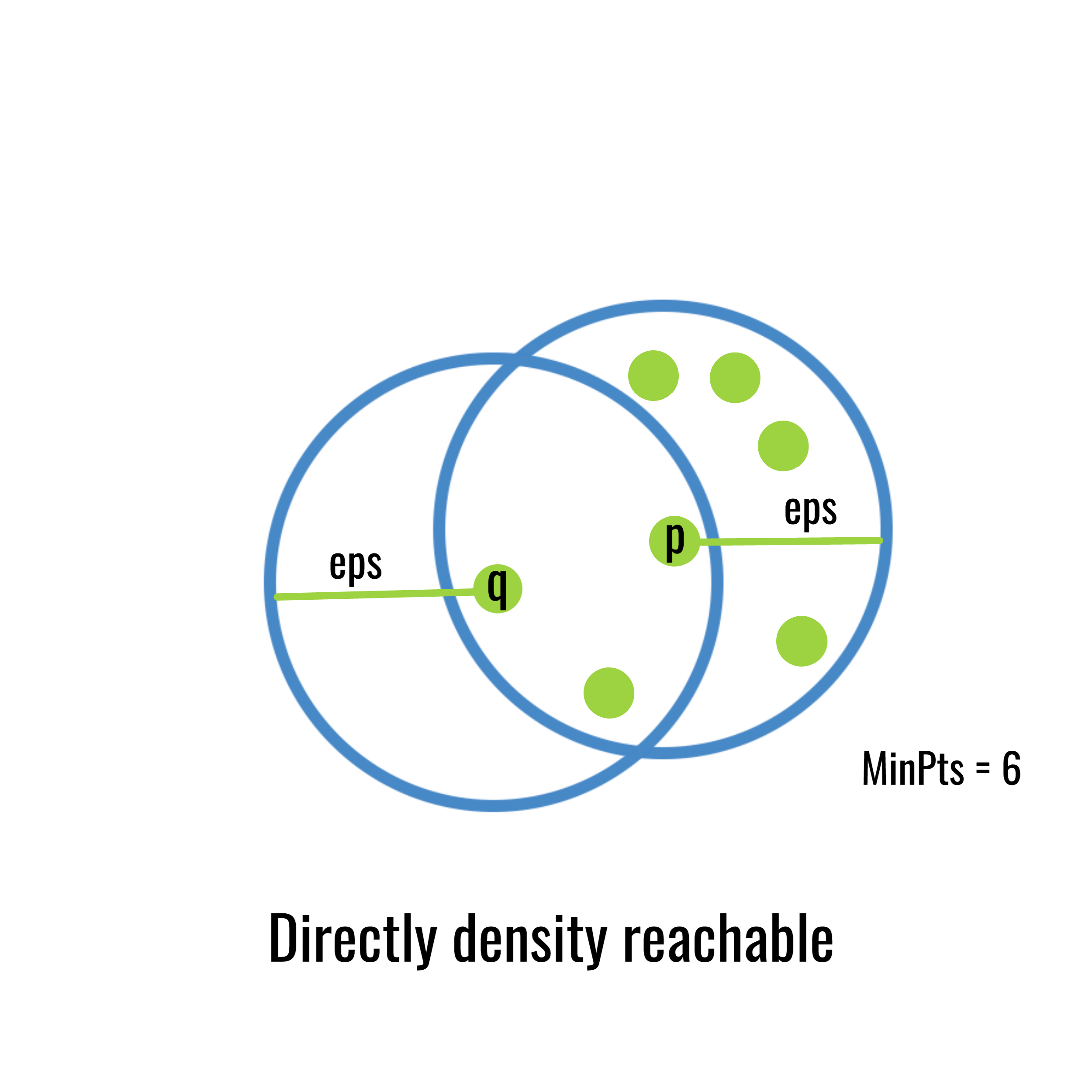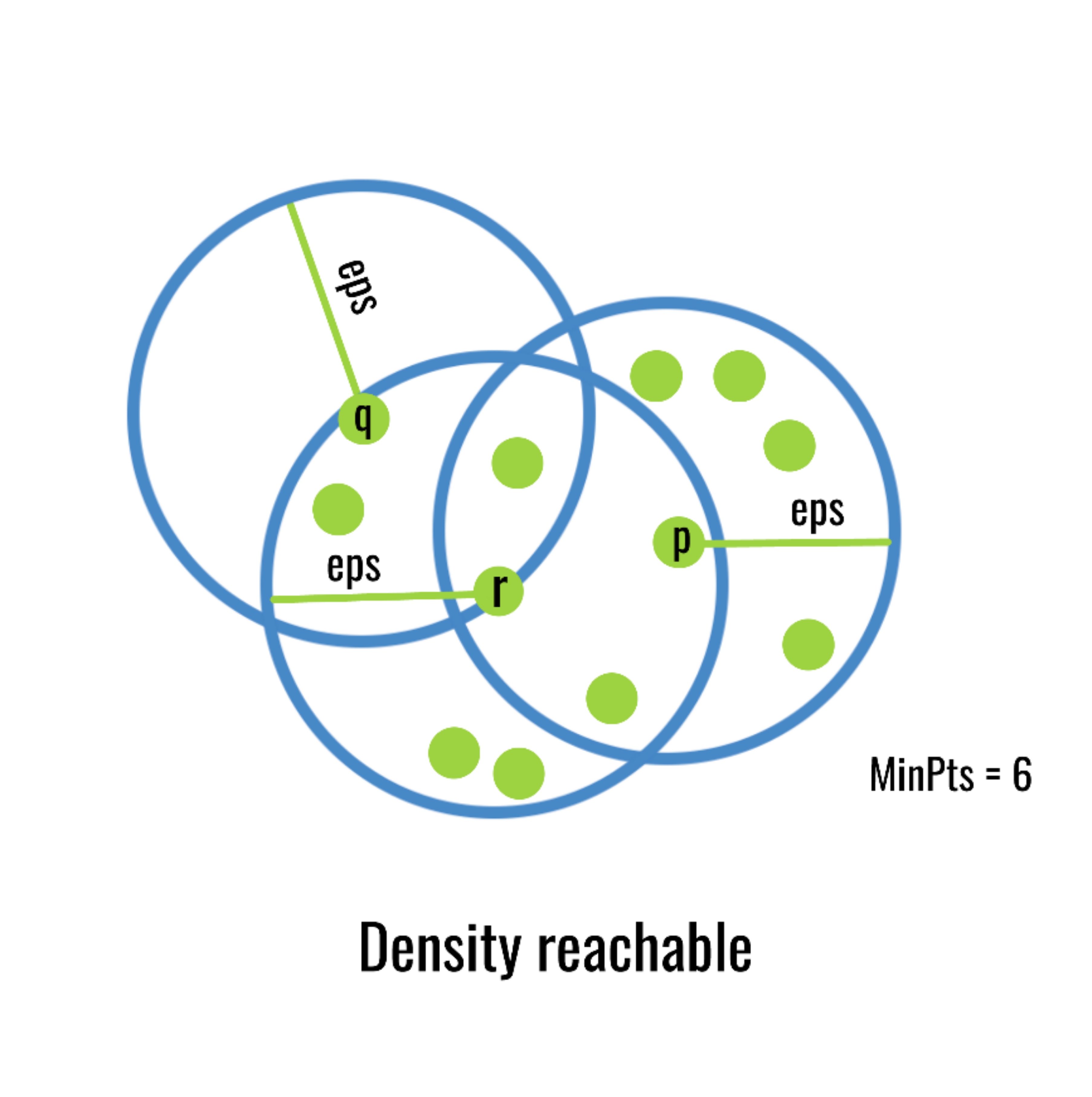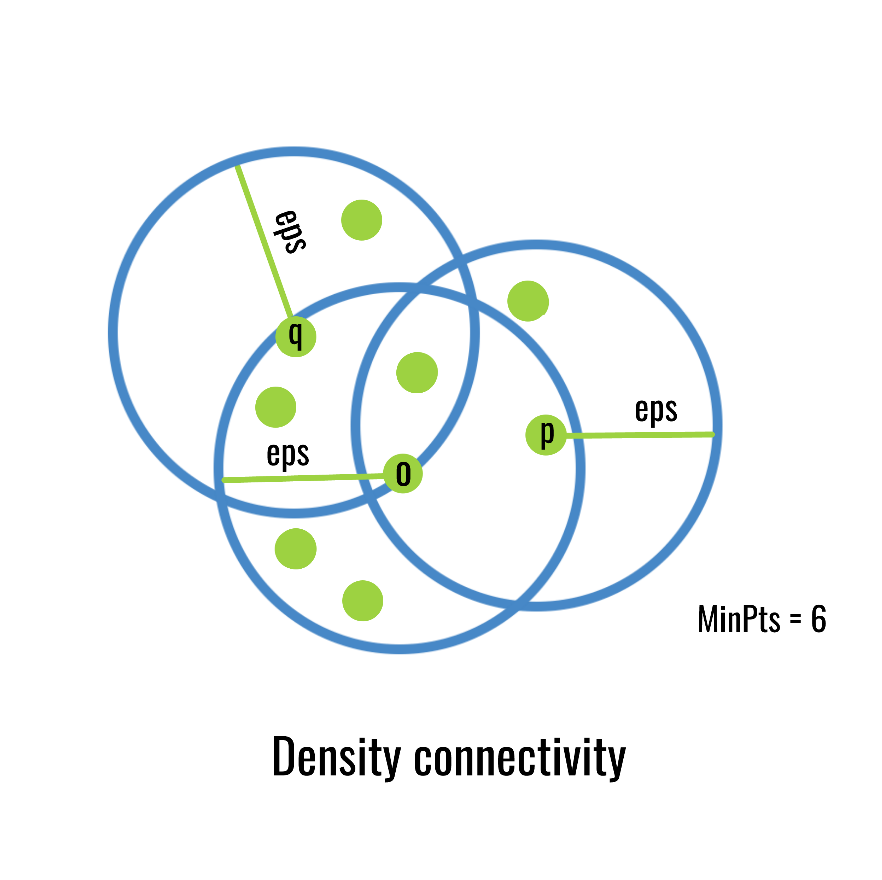Related Articles
ML | DBSCAN reachability and connectivity
• Last Updated : 20 May, 2019

Prerequisite : DBSCAN Clustering in ML

Density-based clustering algorithm has played a vital role in finding nonlinear shapes structure based on the density. Density-Based Spatial Clustering of Applications with Noise (DBSCAN) is the most widely used density-based algorithm. It uses the concept of density reachability and density connectivity.

Consider a set of points in some space to be clustered using DBSCAN clustering. Let ε be the radius of a neighborhood with respect to some point and core objects are the objects whose ε-neighborhood contains at least MinPts number of objects.

### Reachability –

• Directly density reachable:
An object (or instance) q is directly density reachable from object p if q is within the ε-Neighborhood of p and p is a core object.Here directly density reachability is not symmetric. Object p is not directly density-reachable from object q as q is not a core object.

• Density reachable:
An object q is density-reachable from p w.r.t ε and MinPts if there is a chain of objects q1, q2…, qn, with q1=p, qn=q such that qi+1 is directly density-reachable from qi w.r.t ε and MinPts for all 1 <= i <= nHere density reachability is not symmetric. As q is not a core point thus qn-1 is not directly density-reachable from q, so object p is not density-reachable from object q.

### Connectivity –

• Density connectivity: Object q is density-connected to object p w.r.t ε and MinPts if there is an object o such that both p and q are density-reachable from o w.r.t ε and MinPts.Here density connectivity is symmetric. If object q is density-connected to object p then object p is also density-connected to object q.

Based on the above two concepts reachability and connectivity we can define the cluster and noise points.

Cluster:
A cluster C w.r.t. ε and MinPts is a non empty subset of D (the whole set of objects or instances) satisfying –

• Maximality: For all objects p, q if p ε C and if q is density-reachable from p w.r.t ε and MinPts then q ε C.
• Connectivity: For all objects p, q ε C, p is density-connected to q and vice-versa w.r.t. ε and MinPts.

Noise:
Objects which are not directly density-reachable from at least one core object are known as Noise points.My Personal Notes arrow_drop_up